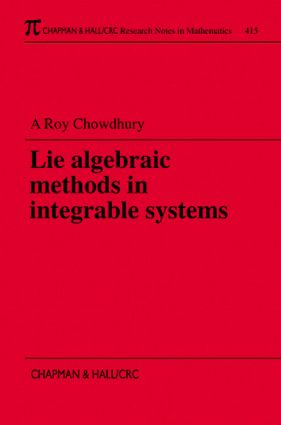# Lie Algebraic Methods in Integrable Systems

## 1st Edition

Chapman and Hall/CRC

368 pages

##### Purchasing Options:\$ = USD
Paperback: 9781584880370
pub: 1999-09-28
SAVE ~\$35.00
\$175.00
\$140.00
x
Hardback: 9781138401921
pub: 2017-07-27
SAVE ~\$41.00
\$205.00
\$164.00
x

FREE Standard Shipping!

### Description

Over the last thirty years, the subject of nonlinear integrable systems has grown into a full-fledged research topic. In the last decade, Lie algebraic methods have grown in importance to various fields of theoretical research and worked to establish close relations between apparently unrelated systems.

The various ideas associated with Lie algebra and Lie groups can be used to form a particularly elegant approach to the properties of nonlinear systems. In this volume, the author exposes the basic techniques of using Lie algebraic concepts to explore the domain of nonlinear integrable systems. His emphasis is not on developing a rigorous mathematical basis, but on using Lie algebraic methods as an effective tool.

The book begins by establishing a practical basis in Lie algebra, including discussions of structure Lie, loop, and Virasor groups, quantum tori and Kac-Moody algebras, and gradation. It then offers a detailed discussion of prolongation structure and its representation theory, the orbit approach-for both finite and infinite dimension Lie algebra. The author also presents the modern approach to symmetries of integrable systems, including important new ideas in symmetry analysis, such as gauge transformations, and the "soldering" approach. He then moves to Hamiltonian structure, where he presents the Drinfeld-Sokolov approach, the Lie algebraic approach, Kupershmidt's approach, Hamiltonian reductions and the Gelfand Dikii formula. He concludes his treatment of Lie algebraic methods with a discussion of the classical r-matrix, its use, and its relations to double Lie algebra and the KP equation.

### Reviews

"Lie theory and algebraic geometry have played a unifying role in integrable theory since its early rebirth some 30 years ago. They have transformed a mosaic of old examples, due to the masters like Hamilton, Jacobi and Kowalewski, and new examples into general methods and statements. The book under review addresses a number of these topics… contains a variety of interesting topics: some are expained in a user-friendly and elementary way, and others are taken directly from research papers."

-Pierre Van Moerbeke, in The London Mathematical Society

### Table of Contents

INTRODUCTION

Lax Equation and IST

Conserved Densities and Hamiltonian Structure

Symmetry Aspects

Observations

LIE ALGEBRA

Introduction

Structure Constants and Basis of Lie Algebra

Lie Groups and Lie Algebra

Representation of a Lie Algebra

Cartan-Killing Form

Roots Space Decomposition

Lie Groups: Finite and Infinite Dimensional

Loop Groups

Virasoro Group

Quantum Tori Algebra

Kac-Moody Algebra

Serre's Approach to Kac-Moody Algebra

Gradation

Other Infinite Dimensional Lie Algebras

PROLONGATION THEORY

Introduction

Sectioning of Forms

The KdV Problem

The Method of the Hall Structure

Prolongation in (2+1) Dimension

Method of Pseudopotentials

Prolongation Structure and the Bäcklund Transformation

Constant Coefficient Ideal

Connections

Morphisms and Prolongation

Principal Prolongation Structure

Prolongations and Isovectors

Vessiot's Approach

Observations

CO-ADJOINT ORBITS

Introduction

The Kac-Moody Algebra

Integrability Theorem: Adler, Kostant, Symes

Superintegrable Systems

Nonlinear Partial Differential Equation

Extended AKS Theorem

Space-Dependent Integrable Equation

The Moment Map

Moment Map in Relation to Integrable Nonlinear Equation

Co-Adjoint Orbit of the Volterra Group

SYMMETRIES OF INTEGRABLE SYSTEMS

Introduction

Lie Point and Lie Bäcklund Symmetry

Lie Bäcklund Transformation

Some New Ideas in Symmetry Analysis

Non-Local Symmetries

Observations

HAMILTONIAN STRUCTURE

Introduction

Drinfeld Sokolob Approach

The Lie Algebraic Approach

Example of Hamiltonian Structure and Reduction

Hamiltonian Reduction in (2+1) Dimension

Hamiltonian Reduction of Drinfeld and Sokolov

Kupershmidt's Approach

Gelfand Dikii Formula

Trace Identity and Hamiltonian Structure

Symmetry and Hamiltonian Structure

CLASSICAL r-MATRIX

Introduction

Double Lie Algebra

Classical r-Matrix

The Use of r-Matrix

The r-Matrix and KP Equation

### Subject Categories

##### BISAC Subject Codes/Headings:
MAT000000
MATHEMATICS / General
MAT002000
MATHEMATICS / Algebra / General
MAT007000
MATHEMATICS / Differential Equations
SCI040000
SCIENCE / Mathematical Physics